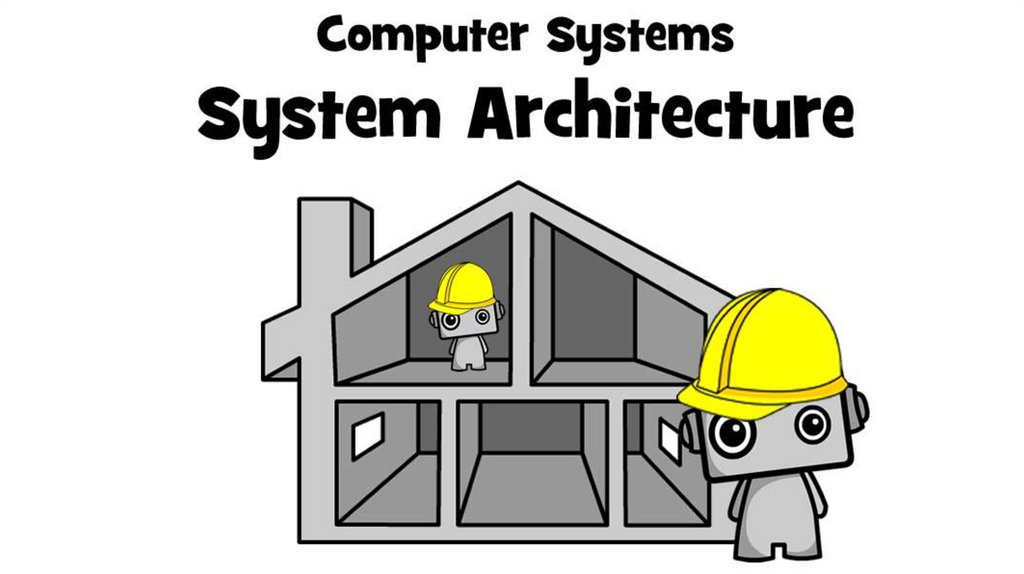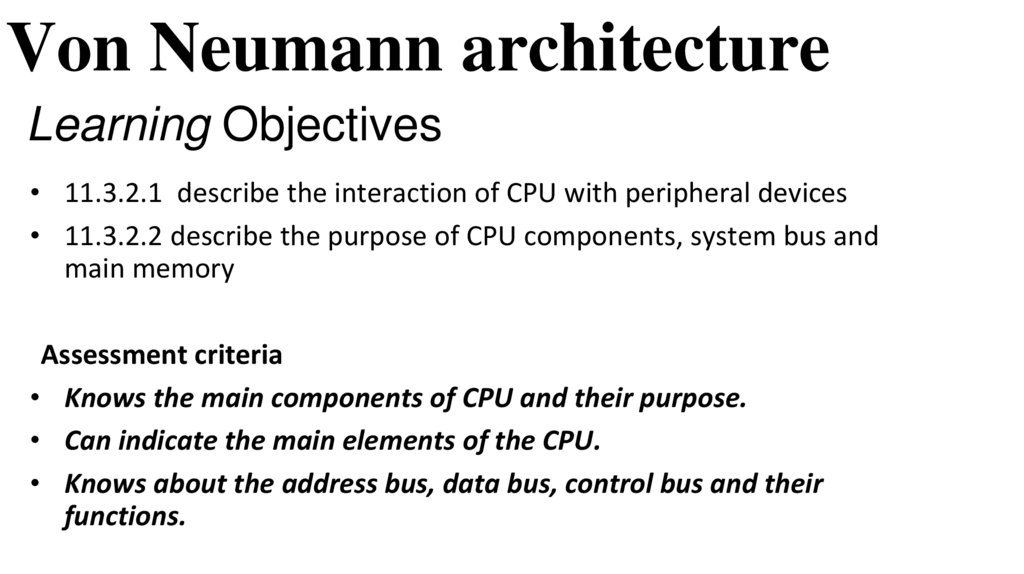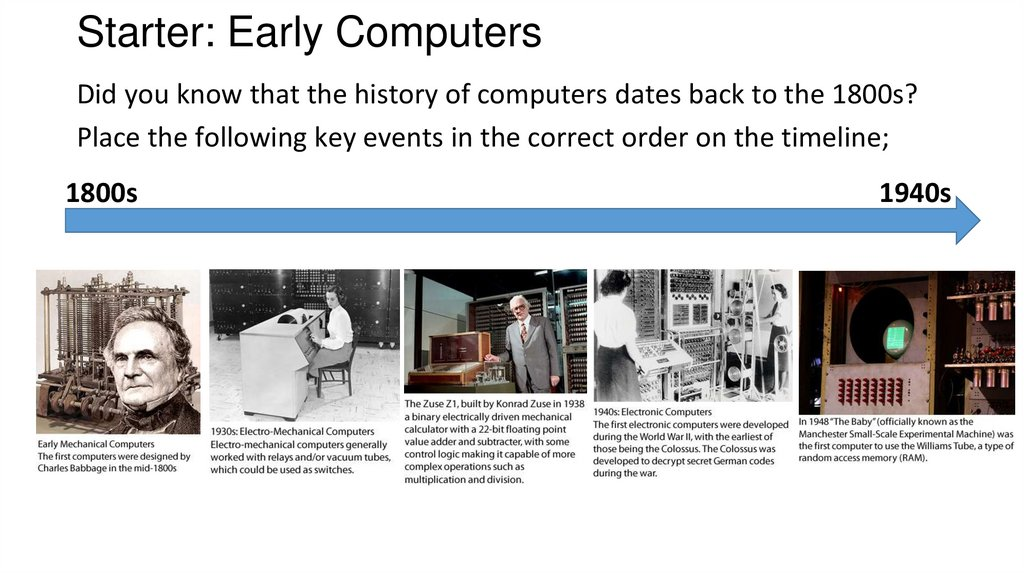# Systems Architecture

## 2. Learning Objectives

Von Neumann architecture
Learning Objectives
• 11.3.2.1 describe the interaction of CPU with peripheral devices
• 11.3.2.2 describe the purpose of CPU components, system bus and
main memory
Assessment criteria
• Knows the main components of CPU and their purpose.
• Can indicate the main elements of the CPU.
• Knows about the address bus, data bus, control bus and their
functions.

## 3. Starter: Early Computers

Did you know that the history of computers dates back to the 1800s?
Place the following key events in the correct order on the timeline;
1800s
1940s

## 4. How Von Neumann Architecture changed the world of computers

• The very first computers could not store
programs; they were input by setting switches.
• Jon Von Neumann developed the concept of
the stored program computer in the 1940s.
• A Von Neumann based computer is one that;
• Uses a single processor.
• Uses one memory for both instructions and data. A
von Neumann computer cannot distinguish
between data and instructions. It ‘knows’ only
because of the location of a particular bit pattern in
RAM.
• Executes programs by doing one instruction after
the next in a sequential manner using a fetchdecode-execute cycle.
http://s7.computerhistory.or
g
All this is only possible through the use of a Central Processing Unit (CPU)

## 5. How does Von Neumann Architecture work?

Watch the video located on this webpage: http://www.bbc.co.uk/education/guides/zmb9mp3/revision/1
Answer the following questions: (Handout 1)
Question
1. What are the key hardware components required within Von Neumann
Architecture?
2. Name the two types of memory used within computers? What are the
characteristics of each type of memory?
3. What is the impact of having more memory available when playing a
computer game?
4. What is the role of an input device? Give an example.
5. Briefly describe what the CPU does.
6. How does the speed of a CPU affect the running of an application e.g. a
computer game?
7. What is the role of an output device? Give an example.

## 6. Von Neumann in detail: Components of the Processor

The processor responds to and
processes the instructions that
drive the computer. It contains the
following components:
• The Arithmetic Logic Unit (ALU)
• Control Unit (CU)
• Clock
• Bus

## 7. The CPU: Arithmetic & Logic Unit (ALU)

The CPU: Arithmetic & Logic Unit (ALU)
The ALU carries out the following
functions:
• Logical operations: Boolean
operations, e.g. AND, OR and NOT
• Shift operations: The bits in a
computer word can be shifted left
or right by a certain number of
places.
• Arithmetic operations: These
multiplication and division.
A word is a fixed-sized piece of data handled as a unit by the
instruction set or the hardware of the processor. Word length
is the number of bits in the internal data path of a processor.

Which of the ALU operations would be best suited for the following
tasks: Logical, Shift or Arithmetic? (handout 2)
Most suitable ALU operation
Checking if an argument is TRUE or FALSE
Subtracting one binary number from another
Multiplying one binary number by another
Checking if an action has repeated a specific number of times
Moving a computer word two places to the left

## 9. Task: ALU or Control Unit? (handout 3)

Place the following statements into the correct category - ALU or Control unit:
Arithmetic & Logic Unit (ALU)
signals from other computer
devices.
as fetching, decoding,
execution handling and
storing results
Control Unit
Some of the logical operations
are as follows: comparison
Interprets instructions
between numbers, letter and or
special characters.
Some
of the operations are
Guides data flow
through
as follows:
different computer
subtraction, multiplication
and division.
Controls sequential instruction execution
Regulates and controls
processor timing
Responsible for the following
conditions: Equal-to conditions,
Less-than condition and greater
Responsible for performing
all logical and arithmetic
operations.

## 10. Task: Von Neumann puzzle (handout 4)

Learners to stick the pieces to create the Von Neumann
Architectural block diagram.
Learners who finish this activity before time should describe
the Von Neumann architecture.